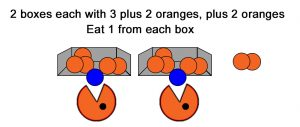# PEDMAS Grouping C

## What’s the Point?

In the real world, all problems start out as word problems that have to be translated in algebra to solve them using math. To get the correct answer if you have an expression with several operations, you have to do them in the standard order.

This is it: the order to do the operations in.

P – Parenthesized blocks first – Evaluate stuff inside parenthesis
E – Exponents next
MD – Multiplication and Division next. Do these in left to right order.
AD – Addition Subtraction last. Do these in left to right order.

## TL;DWIn this example, there are two boxes, each with a group of 3 oranges and a group of 2 oranges. Additionally there are 2 oranges not in any box. Then one orange is eaten from each box. (The blue oranges are the ones being eaten, they get subtracted since they’re actually negative oranges).

A) As always, set up an algebraic expression that represents the problem.

2(3 + 2 – 1) + 2

Here the boxes are represented by the parenthesized expression. Each box had one group of 3, plus one group of 2, and then the negative 1 because one is eaten.

Because there are two of boxes, it’s multiplied by 2. We can do this because the boxes are identical so even though there are two boxes, they can be represented by one expression. However, because there are two of them, we must multiply the entire expression by 2. There’s no times sign because it’s implied by the 2 followed by the parenthesis.

The final +2 is for the last two oranges that aren’t in the boxes.

B) Now solve the problem by simplifying the expression. First, remember PEDMAS. The P stands for parenthesis, so first simplify everything inside the parenthesis before doing anything else.

2(3 + 2 – 1) + 2 = 2 X 4 + 2

Remember that we’re multiplying the parenthesized expression by 2 (because there are 2 boxes), so when we write the simplified expression, we have to write the times sign. It’s no longer implied.

C) Now we finish to get the answer, 10, which is how many are left after the eating one from each box. Again, remember PEDMAS. The M means multiplication and it comes before the A for addition. So, first do the multiply, then do the add.

2 X 4 + 2 = 8 + 2 = 10

Khan has lots more!